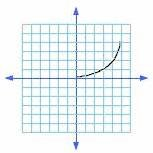## Definition Of Increasing Function

A function f is said to be increasing if f(a) > f(b) when a > b.

The graph of an increasing function always goes up from left to right.
A function is increasing at all the points where its derivative is positive.

### Example of Increasing FunctionIn figure, the curve is going up from left to right. Hence, the function is said to be increasing.

### Solved Example on Increasing Function

#### Ques: Find the interval in which f(x) = 3x? - 54x2 + 14 increases.

##### Choices:

A. (- 3, 3)
B. (0, 8)
C. (0, 3) U (3, 8)
D. (- 3, 0) U (3, 8)